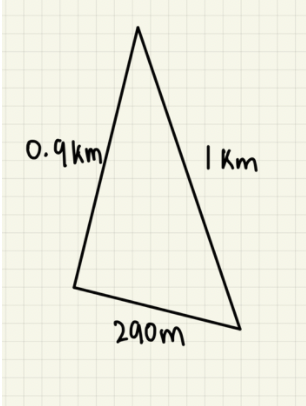# Perimeter

Calculate and understand what the perimeter of a shape means.

The perimeter of the shape is value which tells you the distance around the outside of a shape. The perimeter can be calculated by adding all the side lengths of the shape together. Finding the perimeter can sometimes be made harder by obscuring some of the side lengths. So, here are three simple steps that can help find the perimeter, regardless of whether the side lengths are evident or not.

Step 1: Determine the shape from the question.

The shape you are trying to find the perimeter of is important.

It is important to understand what shape you are dealing with. It is helpful to recognise properties of shapes like: symmetry, side lengths, and angles. For example:This triangle has symmetry around the centre of the shape:This shape has two identical side lengths, which are 12. And, given the isometric triangle shape, we can also determine that the two bottom angles are identical.Step 2: Understand what values you are missing

Once we have determined the shape, and its properties, we can then determine how to use the shapes properties to find missing values. For example:In this shape, we are only given a single side length. We are missing three side lengths. However, knowing that the shape is a square means that we know all the sides are exactly the same length. Therefore, we know this square has 4 sides of length 5.

Step 3: Ensure all the side lengths are in the same units

Questions can sometimes trick you by providing side lengths in different units. For example:Therefore, it is important to put all the side lengths in the same units before adding all the side lengths.

##### Explore Further
Topic Breakdown
Step-by-Step Solutions
Group Tutorial
Individual Assitance